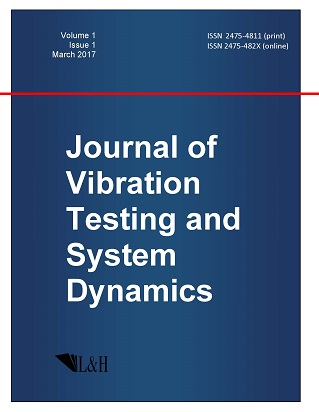ISSN: 2475-4811 (print)
ISSN: 2475-482X (online)
Journal of Vibration Testing and System Dynamics

C. Steve Suh (editor), Pawel Olejnik (editor),

Xianguo Tuo (editor)

Pawel Olejnik (editor)

Lodz University of Technology, Poland

Email: pawel.olejnik@p.lodz.pl

C. Steve Suh (editor)

Texas A&M University, USA

Email: ssuh@tamu.edu

Xiangguo Tuo (editor)

Sichuan University of Science and Engineering, China

Email: tuoxianguo@suse.edu.cn

Inverse Problem for Degenerate Lotka-Volterra System of Three Equations

Journal of Vcibration Testing and System Dynamics 2(3) (2018) 291--296 | DOI:10.5890/JVTSD.2018.09.009

Varadharaj Dinakar$^{1}$, Krishnan Balachandran$^{2}$

$^{1}$ Department of Mathematics, Central University of Tamilnadu, Thiruvarur, India - 610101

$^{2}$ Department of Mathematics, Bharathiar University, Coimbatore, India - 641046

Abstract

We consider the degenerate Lotka-Volterra system with three equations in the linearized form. The internal observations with two measurements are allowed to obtain the stability result for the inverse problem consisting of simultaneously retrieving three coefficients in the given parabolic system with the help of Carleman estimates for the degenerate Lotka-Volterra system.

References

1.   Grammaticos, B., Moulin-Ollagnier, J., Ramani, A., Strelcyn, J.M., and Wojciechowski, S. (1990), Integrals of quadratic ordinary differential equations in R3: The Lotka-Volterra system, Physica A: Statistical Mechanics and its Applications, 163, 683-722.
2.   Hofbauer, J. and Sigmund, K. (1998), The Theory of Evolution and Dynamical Systems, Cambridge University Press, Cambridge.
3.   Pao, C.V. (1992), Nonlinear Parabolic and Elliptic Equations, Plenum: New York.
4.   Pao, C.V. (2004), Global Asymptotic stability of Lotka-Volterra competition systems with diffusion and time delays, Nonlinear Analysis: Real World Applications, 5, 91-104.
5.   Sakthivel, K., Baranibalan, N., Kim, J.H., and Balachandran, K. (2010), Stability of diffusion coefficients in an inverse problem for the Lotka-Volterra competition system, Acta Applicandae Mathematicae, 111, 129-147.
6.   Cannarsa, P. and Teresa, L.D. (2009), Controllability of 1-D coupled degenerate parabolic equations, Electronic Journal of Differential Equations, 2009(73), 1-21.
7.   Cannarsa, P., Martinez, P., and Vancostenoble, J. (2005), Null controllability of degenerate heat equations, Advances in Differential Equations, 10, 153-190.
8.   Cannarsa, P., Martinez, P., and Vancostenoble, J. (2008), Carleman estimate for a class of degenerate parabolic operators, SIAM Journal on Control and Optimization, 47, 1-19.
9.   Du, R. and Xu, F. (2017), Null controllability of a coupled degenerate system with the first order terms, Journal of Dynamical and Control Systems, DOI:10.1007/s10883-016-9353-4.
10.   Mercado, A., Osses, A., and Rosier, L., Inverse problems for the Schröinger equation via Carleman inequalities with degenerate weights, Inverse Problems, 015017.
11.   Cannarsa, P., Tort, J., and Yamamoto, M. (2010), Determination of source terms in a degenerate parabolic equation, Inverse Problems, 26, 105003 (20pp).
12.   Boutaayamou, I., Hajjaj, A., and Maniar, L. (2014), Lipschitz stability for degenerate parabolic sysytems, Electronic Journal of Differential Equations, 2014(149), 1-15.
13.   Boutaayamou, I., Fragnelli, G., and Maniar, L. (2016), Inverse problems for parabolic equations with interior degeneracy and Neumann boundary conditions, Journal of Inverse and Ill-posed Problems, 24(3), 275-292.
14.   Dinakar, V., Baranibalan, N., and Balachandran, K. (2017), Identification of source terms in a coupled age-structured population model with discontinuous diffusion coefficients, AIMS Mathematics, 2, 81-95.
15.   Cristofol, M., Gaitan, P., Ramoul, H., and Yamamoto, M. (2011), Identification of two coefficients with data of one component for a nonlinear parabolic system, Applicable Analysis, 1-9.
16.   Deng, Z.C. and Yang, L. (2011), An inverse problem of identifying the coefficient of first-order in a degenerate parabolic equation, Journal of Computational and Applied Mathematics, 235, 4404-4417.
17.   Evans, L.C. (1998), Partial Differential Equations, AMS, Providence.